Shear Stress in Beams

Consider a segment of the beam shown. The shear load on the vertical surfaces are generated by shear stress that can be calculated by the following process.To calculate the shear stress t generated from the shear load V consider removing the segment of the beam shown in red.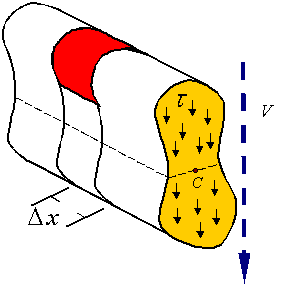By symmetry of stress, shear stresses on the cross section results in equal shear stresses on the plane perpendicular to the cross section as shown. This shear stress results in a shear load Fs.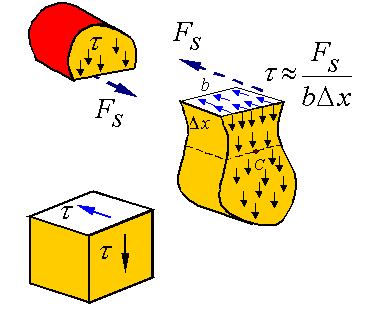The side view of this segment, showing only axial loads, will look as follows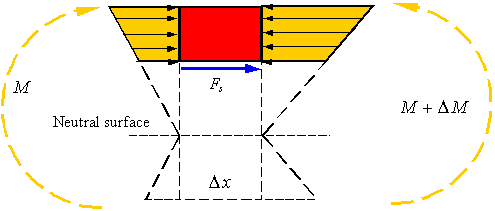As can be seen, the difference in the bending moment on the two sides of the segment results in the normal stresses being different. The shear load Fs is the element that brings the segment into equilibrium. The axial load due to the normal stresses created by the bending moment can be calculated by integrating the normal stress over the area A* of the cross section.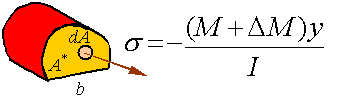Therefore, equilibrium in the axial direction for this segment is written as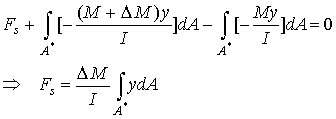The integral in this expression is the first moment of the area A* about the neutral axis. This first moment will be denoted by Q so that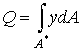The shear stress can now be calculated from the shear load by dividing it by the area it is applied on to get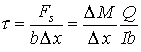Taking the limit as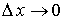giveswhere we note that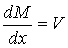Calculating the first moment of the area: The first moment of the area can be calculated from the relationwhere A* is the area of the part of the cross section that is considered,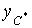is the vertical distance from the centroid of the cross section to the centroid of A*.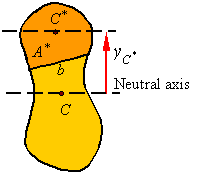For composite areas, the first moment of area can be calculated for each part and then added together. The equation for Q in this case is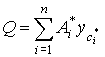Shear stress along a slanted direction: The procedure presented does not require that the segment be cut with a surface parallel to the neutral surface. Therefore, one can remove a segment with the lower surface being at an oblique angle to the neutral surface so that the cross section will look as shown.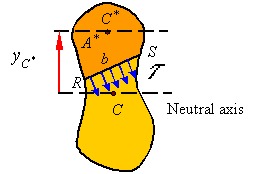The shear calculated in this way is the average shear stress perpendicular to the line RS of width b.